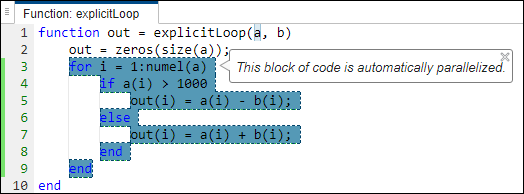## Automatically Parallelize `for` Loops in Generated Code

Iterations of parallel `for` loops can run simultaneously on multiple cores on the target hardware. Parallelization of a section of code might significantly improve the execution speed of the generated code. See How parfor-Loops Improve Execution Speed.

While generating C/C++ code from your MATLAB® code, you can generate parallel `for` loops automatically. Automatic parallelization is a compiler transformation that converts sequential code to multithreaded code without manual intervention.

Automatic parallelization of `for` loop supports these build types for C/C++ targets:

• MEX

• Static library

• Dynamically linked library

• Executable

### Parallelize `for` Loops by Using MATLAB Coder App

To enable automatic parallelization of `for` loops, in the MATLAB Coder™ app, in the Generate Code step, select More Settings > Speed > Enable automatic parallelization.### Parallelize `for` Loops at Command Line

You can enable parallelization of the `for` loops by using the command-line interface. Consider the function `autoparExample`:

```function x = autoparExample(x) %#codegen for i = 10:numel(x) x(i) = sqrt(x(i)); end end```

To automatically generate parallel `for` loops, run these commands:

```cfg = coder.config('lib'); cfg.EnableAutoParallelization = 1; x = rand(1,2000); codegen -config cfg autoparExample -args {x} -report```
`Code generation successful: View report`

### Inspect Generated Code and Code Insights

Open and inspect the code generation report.

#### Generated Code

Observe the Open Multiprocessing (OpenMP) pragmas generated above the `for` loops.

```void autoparExample(double x) { int i; if (!isInitialized_autoparExample) { autoparExample_initialize(); } #pragma omp parallel for num_threads(omp_get_max_threads()) private(i) for (i = 0; i < 1991; i++) { x[i + 9] = sqrt(x[i + 9]); } }```

The gutter highlighted in green next to the loops shows the part of the code that is parallelized.#### Code Insights

In the Code Insights tab, under Automatic parallelization issues, you can see detailed information about the `for` loops that were not parallelized in the generated code.

For example, to view a particular code insight, regenerate code for the `autoparExample` function that you defined in the previous section. Specify a smaller size for the input arguments.

```cfg = coder.config('lib'); cfg.EnableAutoParallelization = 1; x = rand(1,1000); codegen -config cfg autoparExample -args {x} -report```

The generated code does not contain parallel `for` loops because the size of the input argument is smaller than the threshold value for automatic parallelization. To view detailed information about the nonparallelized part of the code, open the report and click Code Insights > Automatic parallelization issues.### Disable Automatic Parallelization of a `for` Loop

You might want to disable automatic parallelization of a particular loop if that loop performs better in serial execution. To prevent parallelization of a specific `for` loop, place the `coder.loop.parallelize('never')` pragma immediately before the loop in your MATLAB code. This pragma overrides the `EnableAutoParallelization` setting. Also, this pragma supports only those `for` loops that are explicitly defined in your MATLAB code. For more information on explicit and implicit loops, see the next section.

For example, the code generator does not parallelize this loop:

```% Pragma to disable automatic parallelization of for-loops coder.loop.parallelize('never'); for i = 1:n y(i) = y(i)*sin(i); end```

### Parallelize Implicit `for` Loops

The example function `autoparExample` used in the previous sections contains an explicit `for` loop. But your MATLAB code can also contain implicit `for` loops that do not appear explicitly in the code that you author. For example, the MATLAB function `mtimes` multiplies two matrices and must perform loop iterations implicitly over the matrix elements.

Automatic parallelization supports loops that are implicit in your MATLAB code. For example, consider this function `autoparExample_implicit`.

```function y = autoparExample_implicit(y) %#codegen y = y * 17; % Generates implicit for loop end```

Generate code by running these commands:

```cfg = coder.config('lib'); cfg.EnableAutoParallelization = 1; y = rand(1,2000); codegen -config cfg autoparExample_implicit -args {y} -report```

Open the report and inspect the generated code. The generated code contains a parallel `for` loop for the multiplication operation.

```void autoparExample_implicit(double y) { int i; if (!isInitialized_autoparExample_implicit) { autoparExample_implicit_initialize(); } #pragma omp parallel for num_threads(omp_get_max_threads()) private(i) for (i = 0; i < 2000; i++) { y[i] *= 17.0; }```

### Parallelize `for` Loops Performing Reduction Operations

You can parallelize `for-`loops performing reduction operations by using the configuration option Optimize reductions.

To enable automatic parallelization of these `for`-loops:

1. Open the MATLAB Coder app.

2. On the Generate Code page, click More Settings.

3. On the Speed tab, select the Enable automatic parallelization and Optimize reductions check boxes.Optimize reductions also gets enabled, if you set the Leverage target hardware instruction set extensions parameter to an instruction set that your processor supports.

To enable the configuration option `OptimizeReductions` by using the command-line interface,run these commands.

```cfg = coder.config('lib'); cfg.EnableAutoParallelization = true; cfg.OptimizeReductions = true;```

For example, write a MATLAB function `arraySum` which calculates and stores the sum of the elements of the array in the reduction variable `out`.

```function out = arraySum(n) %#codegen in = ones(n,n) out = 0; for i = 1: out = out + in(i) + i; % reduction operation end end ```

At the MATLAB command line, run this `codegen` command.

`codegen arraySum -config cfg -args {5} -report`
`Code generation successful: View report`

Open the code generation report by clicking View report, to see the parallelized `for`-loop for the addition operation.

```#pragma omp parallel num_threads(omp_get_max_threads()) private(outPrime) { outPrime = 0.0; #pragma omp for nowait for (b_i = 0; b_i < i1; b_i++) { outPrime = (outPrime + 1.0) + ((double)b_i + 1.0); } omp_set_nest_lock(&xAddition_nestLockGlobal); { out += outPrime; } omp_unset_nest_lock(&xAddition_nestLockGlobal); }```

### Usage Notes and Limitations

• Empty loops and `while` loops are not parallelized automatically

• Only the arithmetic reduction operations addition (+), subtraction (-), and product (*) support automatic parallelization in code generation.